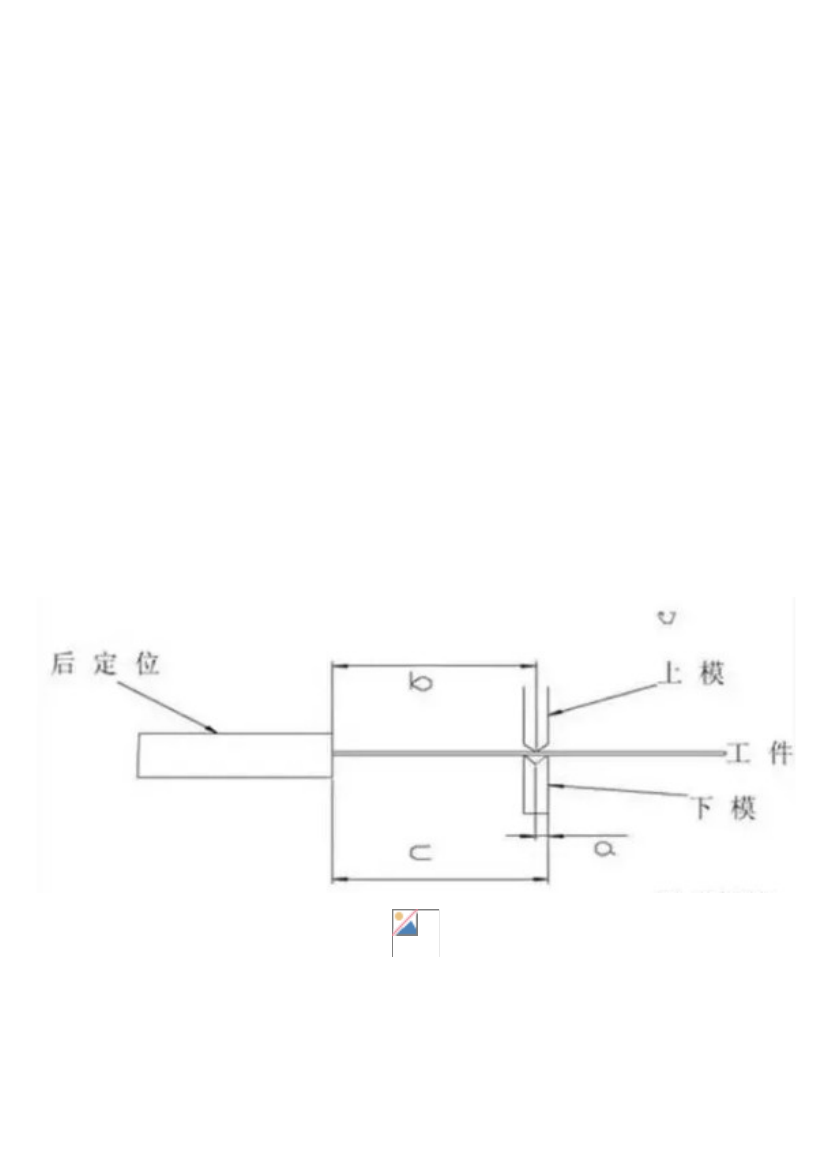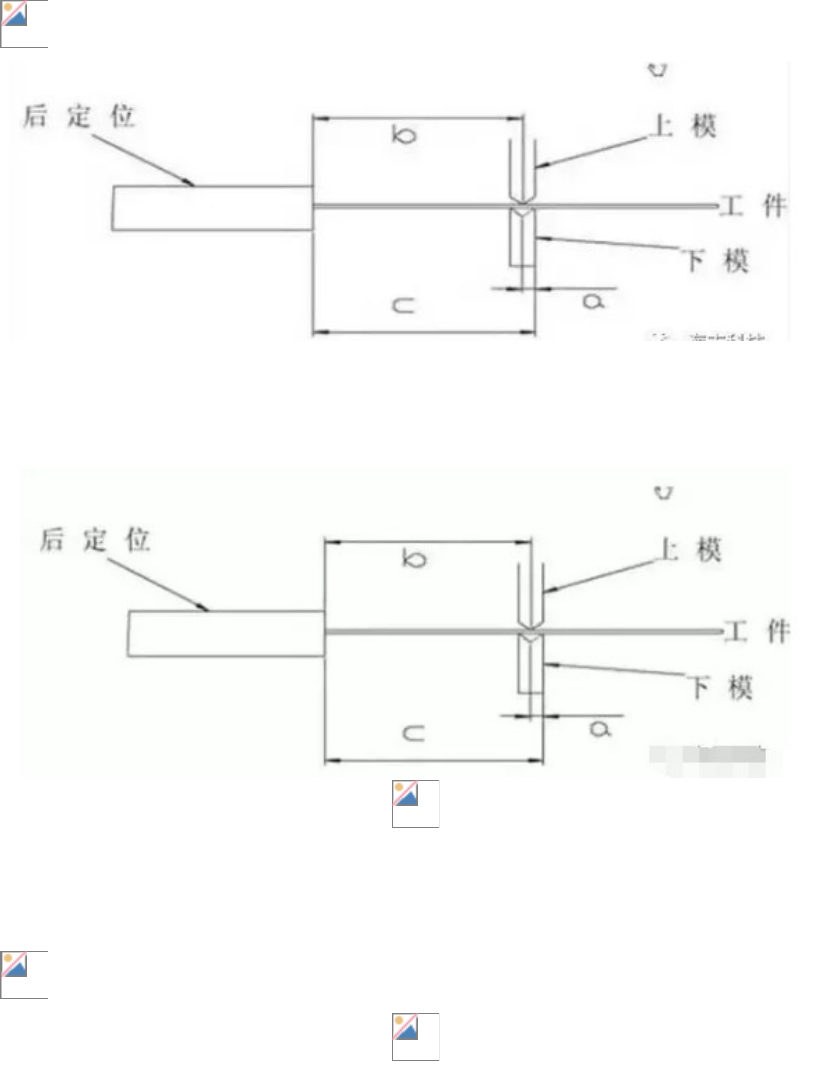# 钣金折弯模具工艺！如何做这三种折弯试模，选择合适方法最重要.doc

1858898****  |   2019-09-24
类型：工艺知识   |   格式：doc   |  总 2页   | 上传时间： 2019-09-24-b=-=b
=10mmb=9.2mm=10-9.2= 0.8mm
=10+0.8=10.8mm 10mm a
0.8mm
1/2
b=c-a=b+ 1/2 +b
t=1 a=7mm c=15mm b=15-7=8mm
=8+0.1+1=9.1mmt=1mm b=9mm
=9+0.1+1=10.1mmb

• / 2

0.15M